```

```
Stereometric Basic forms of Inorganic Beings
```
```
Promorphology of Crystals

preparation

3-D Crystals
II

```

```
REMARK : When the reader feels satisfied with our established theoretical foundation (done in previous documents) that allows (single, non-twinned) Crystals to be promorphologically assessed, he or she can skip all the following, and directly proceed with The Promorphology of Crystals.
```
```
Deriving the Complex Motif of Halite (NaCl)

The structure of crystals of Sodium Chloride ( NaCl , Common Salt), which occur in Nature as the mineral Halite, is relatively easy to understand. It consists of two species of chemical element, Sodium and Chlorine. In the crystal these elements occur as ions, i.e. as electrically charged atoms. The Sodium ions bear one positive charge, while the Chlorine ions bear one negative charge. Knowing that opposite electrical charges attract one another, this means that each Sodium ion wants to have as many Chlorine ions around it as possibe, and each Chlorine ion wants to have as many Sodium ions around as possible. And in the present case of NaCl the ions attract or repel one another indiscriminately, i.e. there are no other forces involved (This in contradistinction to, for example, Zinc Sulfide, where, in addition to these electrostatical forces also other forces are at work, in the form of chemical bonding. Because of this the ions decide to stand away from one another at definite angles, causing a different structure).

The point symmetry of crystals of Sodium Chloride is 4/m 3* 2/m. In this symbol "4" stands for the presence of 4-fold rotation axes, "m" stands for mirror planes, "2" stands for 2-fold rotation axes, and " 3* " stands for 3-fold rotoinversion axes. "4/m" stands for the fact that each 4-fold axis has a mirror plane perpendicular to it, while "2/m" stands for the fact that each 2-fold axis has a mirror plane perpendicular to it.
This point symmetry assigns such a crystal to the Hexakisoctahedric Class of the Isometric Crystal System (also called the Cubic Crystal System).
The Space Group ( =  point symmetry + all translational symmetries) of Sodium Chloride is F 4/m 3* 2/m.
The NaCl structure is based on a Cubic Face-centered Point Lattice, indicated by the letter F in the Space Group symbol. Such a lattice is one of the Bravais lattices. Recall that in 3-dimensional space there are only 14 unique patterns of periodically repeated identical points, such that the surroundings of every point of such a pattern are identical. These 14 point patterns are the 14 Bravais lattices.
A cubic face-centered point lattice is one of these patterns. Its unit cell has the form of a cube, and, in addition to its having points at the cube's vertices (corners), it has also points at the centers of each cube face. This cubic face-centered lattice, which forms the basis of the structure of NaCl crystals, is depicted in the next Figure.Figure 1.   (Unit cell of) the Cubic Face-centered Point Lattice, lying at the base of the NaCl structure. The points of the lattice are indicated by black discs. Depicted is a unit cell. Enless repetition of this unit cell in three dimensions yields the point lattice.

```
```
The points of such a lattice can be associated with chemical motifs. When the motifs are equivalent with respect to each other, the structure not only has received content but remains (as was the point lattice) periodic. We could, for example, associate the lattice points with Chlorine ions. Or we could associate these points with Sodium ions.
Well, the NaCl structure consists of two interpenetrating cubic face-centered lattices. These two lattices are shifted with respect to one another by half a body diagonal (i.e. the line connecting two opposite corners of the unit cell cube). One of these lattices has its points associated with Chlorine ions, the other lattice has its points associated with Sodium ions. The structure -- the NaCl crystal structure -- that results is depicted in the next Figure.Figure 2.   ( Unit cell of ) the NaCl structure. It consists of two interpenetrating cubic face-centered lattices, one with Chlorine ions, the other with Sodium ions. The lattices are shifted by 1/2 a body diagonal of the (cubic) unit cell. Larger blue spheres represent Chlorine ions. Smaller red spheres represent Sodium ions. The Sodium ions and the Chlorine ions attract each other uniformly. This means that not only the spheres must be imagined to be contiguous, but also that each ion has six nearest neighbors of the opposite sign resulting in the present structure.

```
```
To understand this structure we must return to the  c l o s e - p a c k e d  s t r u c t u r e s  again, as we've spoken of in the previous document. A close-packed structure is to be expected only in crystals made of a single kind of atoms. And those atoms must not combine with one another in strong chemical bonds to form molecules (also not those consisting of the same kind of atoms), molecules in which the atoms are tightly connected in pairs or rings or chains. Two classes of substances fill these requirements :   the metals and the "rare gases" (inert gases) such as Argon and Neon. In fact, both classes furnish many examples, the metals at ordinary temperatures and the rare gases when they are solidified at very low temperatures.
There are several ways to create a close-packed structure. One way was discussed in the previous document, namely the AB-structure (Hexagonal close-packing). This structure emerges when we start with a layer of contiguous identical spheres, as in the next Figure.Figure 3.   A layer of close-packed identical spheres. With "close-packed" we always mean "packed together as close as possible".

```
```
This first layer we call the A-layer. When we want to add a second layer which is itself also close-packed and which is as close to the first layer as possible, we must put the spheres of this second layer -- which we call the B-layer -- in dimples of the first layer. Only half of these dimples can be used, as the next two Figures illustrate :Figure 4.   A layer of close-packed identical spheres.
Only half of these dimples can accomodate spheres of a second layer, either the ones represented by a red color, or those represented by a white color.

```
```Figure 5.   A second layer of close-packed identical spheres (B) is laid over the first layer (A). In this case the "red" dimples accomodate the spheres of the second layer (the structure will not be different when we had used the "white" dimples).

```
```
When we now add a third layer, we have two possibilities :   either the spheres of this third layer come to lie exactly above those of the first layer, or they do not. In the first case the positions of the spheres of the third layer correspond exactly with those of the first, so we can call the third layer A again. When we, when keeping on stacking layers of identical spheres, repeat this sequence, we get the AB-structure discussed in the previous document.
When on the other hand, we put the third layer such that its spheres do not come to lie exactly above those of the first layer, but above dimples of it, we have a new, i.e. third, position of the spheres of the third layer. Accordingly we will call this third layer the C-layer. When we, when keeping on stacking layers of identical spheres, repeat this sequence, we get the so-called ABC-structure. Its layers are stacked according to the sequence ABCABCABC ... etc. Also this is a close-packed structure of identical spheres, but its inherent symmetry is different from the AB-structure. The next Figure depicts a possible unit cell of the ABC-structure.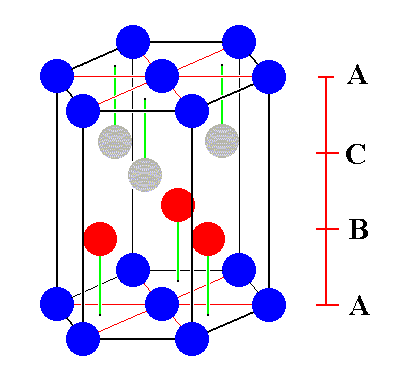Figure 6.   A possible unit cell of the ABC close-packed structure of identical spheres. The shape is a regular hexagonal prism. Also one third of this cell could serve as a unit cell. Its shape would then be that of a rhombic prism.

```
```
If, in the above given unit cell, we extend the B-layer with three more spheres, and also extend the C-layer with three more spheres, fully in accord with the pattern of the ABC-stucture, then we get the following :Figure 7.   The B-layer and the C-layer of the unit cell of the ABC close-packed structure of identical spheres are each extended with three more spheres.
[ Notice that of the spheres belonging to the bottom face of the hexagonal prism, the left back one is masked by one of the newly added spheres in the B-layer. So after the addition of some spheres we still have three layers :   The A-layer (dark blue spheres of the bottom and top faces of the hexagonal prism), then the B-layer (red spheres), and then the C-layer (grey spheres).]
The inscribed hexagons (yellow) clearly show the stacking mode of the ABC-structure.

```
```
The next Figure depicts the same situation as in Figure 7, but now emphasizes the three added spheres in the B-layer : they are colored light blue. It also emphasizes the three added spheres in the C-layer : they are colored green.Figure 8.   Emphasizing the added spheres in the B-layer (light blue), and the added spheres in the C-layer (green) of the unit cell of the ABC close-packed structure of identical spheres. Of the spheres belonging to the base of the hexagonal prism, the left back one happens to be masked by a newly added sphere (light blue) in the B-layer. So this light blue sphere does not belong to the A-level, but to the B-level.

```
```
After we have thus added these extra spheres to the ABC-structure, i.e. after we have extended it according to its inherent pattern, we discover that we can inscribe a face-centered cube. This is a cube with spheres (representing lattice points) at all its corners as well as with spheres (also representing lattice points) in the center of each of its faces. This cube can be seen as an alternative unit cell of the ABC-structure. See next Figure.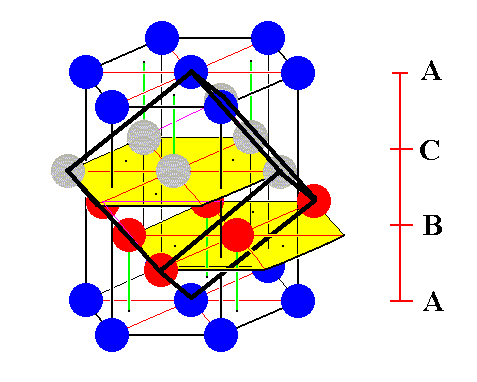Figure 9.   Addition of some more spheres to the ABC-structure in accordance with its inherent pattern, reveals a face-centered cube (indicating by the strong black lines) that can equally well serve as a unit cell of the ABC-structure.

```
```
Let's dig out this cube.Figure 10.   A first stage in (the process of) isolating the face-centered cubic unit cell.

```
```Figure 11.   A second stage in (the process of) isolating the face-centered cubic unit cell.

```
```Figure 12.   A third stage in (the process of) isolating the face-centered cubic unit cell.

```
```Figure 13.   Last stage in (the process of) isolating the face-centered cubic unit cell. The spheres that lie at the corners of the cube are indicated in red. The other colors indicate spheres that lie in the center of each cube face. The green sphere is centering the cube's face that is directed to the observer (front face), while the light blue sphere is centering the back face of the cube. All the faces of the cube are, however, fully equivalent, and the same goes for all the spheres. The next Figure emphasizes this fact.

```
```Figure 14.   A face-centered cubic unit cell of the ABC-structure.

```
```
Because the ABC-structure admits of a  c u b i c  unit cell, it must belong to the Cubic Crystal System (also called Isometric System), and not, as was the case with the AB-structure, to the Hexagonal Crystal System. That's why we call the ABC-structure Cubic Close-packed. Of course we must imagine all the spheres in all the above Figures to be contiguous. We had built the ABC-structure by means of stacking layers of identical spheres, a stacking in a certain direction. But because the ABC-structure is cubic we can consider it at the same time as being stacked in the other three equivalent directions as well. These directions are parallel to the cube's body diagonals. So when you have built the structure, there is no way of telling from the finished structure that you stacked the layers perpendicular to a particular one of those diagonals, because the latter are wholly equivalent. You could have built the structure equally well by stacking the layers perpendicular to any of the four diagonals (these are the four axes of 3-fold symmetry).
Crystals of the metal Copper possess this Cubic Close-packed Structure (ABC-structure).

Now it is time to return to the NaCl crystal structure. This structure consists of two different kinds of chemical motifs, Sodium ions and Chlorine ions. These ions differ in size, or at least they behave as if they were of different size. So the structure as such cannot be an ABC close-packed structure. But if we look at the  o r d e r i n g  of either the Sodium ions or of the Chlorine ions, then we see that each of them is ordered according to a face-centered cubic lattice. So qua  ordering the Sodium ions have arranged themselves according to the ABC-structure, and also the Chlorine ions are ordered according to the ABC-structure. Indeed the NaCl-structure can be seen as two interpenetrating face-centered cubic lattices. Those lattices are shifted with respect to one another by half a body diagonal of a face-centered cubic unit cell. To show this we repeat a Figure given earlier.Figure 15 (= Figure2).   ( Unit cell of ) the NaCl structure. It consists of two interpenetrating cubic face-centered lattices, one with Chlorine ions, the other with Sodium ions. The lattices are shifted by 1/2 a body diagonal of the (cubic) unit cell. Larger blue spheres represent Chlorine ions. Smaller red spheres represent Sodium ions. The Sodium ions and the Chlorine ions attract each other uniformly. This means that each ion has six nearest neighbors of the opposite sign resulting in the present structure, and that the spheres must be imagined to be contiguous. Ions of the same sign are not contiguous.

```
```
Of course the cubic unit cell can also be chosen (i.e. isolated from the NaCl lattice) such that the Sodium ions (instead of the Chlorine ions) come to lie at its corners. See next Figure.Figure 15a.   ( Unit cell of ) the NaCl structure. It consists of two interpenetrating cubic face-centered lattices, one with Chlorine ions, the other with Sodium ions. The lattices are shifted by 1/2 a body diagonal of the (cubic) unit cell. Larger blue spheres represent Chlorine ions. Smaller red spheres represent Sodium ions.

```
```
The whole NaCl structure (i.e. not only its unit cell) contains a  w h o l e  p e r i o d i c  p a t t e r n  of the symmetry elements of the unit cell. Every element of this pattern contains the complete set of these symmetry elements. When a NaCl crystal starts to grow from a microscopical seed containing one set, it will, when growing undisturbed in a uniform medium, develop into a crystal possessing, as a whole, this set of symmetry elements (representing its point symmetry), because every symmetry element present in the lattice structure involves the whole lattice, not only the chemical motifs that lie in the immediate vicinity of such a symmetry element.
These matters can also be expressed in a somewhat differrent way :
If the observer imagines himself to be of only molecular dimensions, then the crystal lattice extends virtually indefinitely for him :   this is the  m i c r o s c o p i c a l  c o n s i d e r a t i o n.  And, that means that, because of the periodic nature of this lattice, there can be a multitude (instead of just one)  p a r a l l e l  symmetry axes (rotation, rotoinversion or screw axes), and also parallel mirror planes and/or glide planes (See for instance Figure 20). These symmetry axes and planes are repeated, i.e. copied, according to the given lattice, resulting in the fact that all the copies of a particular symmetry axis or plane have the same orientation. However, as soon as one  c o n s i d e r s  the crystal  m a c r o s c o p i c a l l y,  i.e. as soon as one sees the crystal as a finite piece of matter, then more than one mirror or glide plane in the same direction (i.e. parallel mirror or glide planes) are impossible. If there is a mirror plane present at all -- and glide planes are turned into mirror planes as soon as the consideration is macroscopic, because the translations are then not visible anymore -- then this mirror plane must go through the  c e n t e r  of the crystal. The same goes for symmetry axes (rotation, etc.) :   if such an axis is present at all, then it goes through the center of the crystal. And if there are more such axes of the same kind, they all go through the center of the crystal, implying that they are never parallel to each other.

As has been said, each lattice resembles the ABC-structure, but only so with respect to the arrangement of the motifs. The latter stand apart from each other (i.e. really stand apart from each other, not only because of a convenience in drawings) :   The Chlorine ions in their face-centered lattice repel each other, and consequently stand away from each other. Likewise the Sodium ions :   they repel each other and consequently want to stand away from each other. But of course the Chlorine ions are, wherever they can, in contact with Sodium ions because they attract each other. They want to be as close as possible to one another.
In the above Figure the Sodium ions are drawn such, as not only standing apart from their fellow Sodium ions, but also from neighboring Chlorine ions. The same is done with respect to the Chlorine ions. The reason for doing so is to let the drawing clearly express the  o r d e r i n g  of the crystal constituents.

Recall that we are trying to determine the Complex Motif of crystals of NaCl. This Complex Motif is the translation-free residue of the NaCl crystal structure. It is the motif that emerges after (conceptually) eliminating all translations inherent in the structure. Because of this, the resulting Complex Motif is not periodic anymore. It has a tectological structure. And on this structure we can base a promorphological assessment of NaCl crystals, i.e. we can determine the crystal's promorph. The latter will finally be represented (by us) by that geometric body that geometrically fully expresses the symmetry content of the Complex Motif.
Of course we know already beforehand what this symmetry content is :   It is equal to the point symmetry of the given crystal on which we base the allocation of that crystal in a certain Crystal Class. Well, NaCl crystallizes in the Hexakisoctahedric Class of the Cubic Crystal System (i.e. in the System's highest symmetrical Class), so its point symmetry is 4/m 3* 2/m. The simplest geometric body expressing this symmetry is the cube. But the cube is idem specie divisible, i.e. it can be divided in eight subcubes which have the same symmetry. So our choice of representing the promorph is expected to be directed to another simple geometrical body, also expressing this symmetry, but not idem specie divisible, namely the regular octahedron. The most compelling reason anyway to choose one geometrical body in favor of another (in the case of them having the same symmetry) is, however, the number of  a n t i m e r s  which the Complex Motif of the crystal possesses, provided that antimers are discernable at all. If the Complex Motif has six antimers, then we must (in spite of the property of idem specie divisibility) assign the crystal promorphologically to the Hexaedra regularia (Polyaxonia). If on the other hand, the Complex Motif possesses eight antimers then the corresponding promorph would be that of the Octaedra regularia (Polyaxonia). The symmetry content of both promorphs is the same, namely 4/m 3* 2/m, but their homotypic number (i.e. their number of antimers) is different. As we will see in due course, the Complex Motif turns out to possess eight antimers, implying that single non-twinned crystals of NaCl must promorphologically be assigned to the Octaedra regularia (Regular Octahedron). Such a body is an endospherical polyhedron possessing eight faces which represent eight antimers.
So what we see here is that for the assessment of the symmetry content of the promorph, we do not necessarily have to isolate the Complex Motif. Only in cases where we end up with a symmetry content that is compatible with several (instead of just one) promorphs -- as we just saw in the case of the 4/m 3* 2/m symmetry which is compatible with the Hexaedra regularia as wel as with the Octaedra regularia -- we must determine the number of antimers, and for this we need the Complex Motif.

This Complex Motif can, as has been said, be derived by eliminating all translational symmetries from the given crystal structure. The elimination of the glide and screw translations transforms glides into mirror planes and screws into ordinary rotation axes, and this normally results in an increase in symmetry (the latter either increases or stays the same).
Well, if we look to the cubic unit cell, as depicted in Figure 15a, we see that it has the highest symmetry (4/m 3* 2/m) that can occur in crystals at all. So from this we know that if the NaCl structure has glides and/or screws, the elimination of their translational elements will not increase the symmetry anymore. So with this cubic unit cell we have already obtained the Complex Motif of crystals of NaCl, and we can assessed the corresponding promorph on the basis of its symmetry and its number of antimers.

The next consideration, however, actually  d e m o n s t r a t e s  that in the case of NaCl the elimination of the glide translations (there are no screw axes present in this structure) does not result in a higher symmetry.

A  s h o r t c u t  to this demonstration is the following :   If we look to the glide planes present in the NaCl structure (See Figure 20 showing this in two dimensions) we see that they are interleaved with mirror planes parallel to them. So the elimination of the translational components of the glide operations leads to their transformation into mirror planes parallel to already existing mirror planes. So these eliminations do not lead to new mirror plans but only to mirror planes parallel to already existing mirror planes. If we descend to the Complex Motif, as the translation-free residue of the lattice structure, then of all these parallel mirror planes only one remains (accommodating for the crystal's center to lie in). So it does not matter that there are, or are not, in addition to existing mirror planes, glide planes parallel to them :   in both cases only one mirror plane survives in the Complex Motif (and in the crystal's point symmetry anyway).Figure 16.   A face of the cubic unit cell of NaCl.
The large blue circles stand for Clorine ions, the smaller red circles stand for Sodium ions. In fact the unit cell is such as depicted in the right image :   Repetition of precisely this configuration yields the NaCl structure, as the next Figure shows.

```
```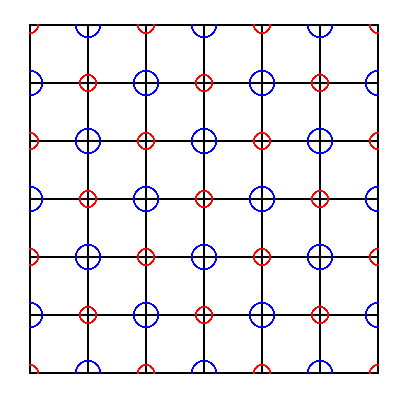Figure 17.   Repetition of the right image of the previous Figure yields the NaCl structure (shown by means of a consideration of one layer (slice) of this structure). The repetition can go on indefinitely.

```
```
The next Figure also represents a repetition (to a somewhat smaller extent), but now with all the ions in tact.Figure 18.   The same as in Figure 17, but now with all the ions intact.

```
```
The next Figures show the inherent glide lines and mirror planes of the two-dimensional slice of the NaCl lattice as depicted in the previous Figure.Figure 19.   Indication of glide lines in a two dimensional slice of the NaCl crystal structure. The slice contains a face of the cubic unit cell. One of the glides is emphasized for clarification. The structure must be imagined to be extended indefinitely.

```
```Figure 20.   Indication of non-diagonal mirror lines in a two dimensional slice of the NaCl crystal structure. The slice contains a face of the cubic unit cell. The structure must be imagined to be extended indefinitely.

```
```Figure 21.   Indication of also the diagonal mirror lines (pink lines) in a two dimensional slice of the NaCl crystal structure. The slice contains a face of the cubic unit cell. In it all glide lines and all mirror lines are indicated. The structure must be imagined to be extended indefinitely.

```
```
The two-dimensional image of Figure 18 can as such represent the NaCl structure, consisting of two interpenetrating face-centered cubic lattices, one furnished with Chlorine ions, the other with Sodium ions. The next Figure depicts (a two-dimensional slice of) the Clorine lattice of NaCl.Figure 22.   A two-dimensional slice of the Chlorine lattice. It is part of the total structure of NaCl. Indicated (yellow) is a face of a unit cell. The arrow represents the distance ( t ) of the simple translations according to which the unit cells are stacked in three dimensions resulting in the periodic structure, the NaCl lattice ( = interpenetration of two cubic face-centered lattices, one with Sodium, one with Chlorine). One glide line ( g ) with respect to the ordering of Chlorine ions is indicated.

```
```
If we conceptually shift every second (i.e. alternative) Chlorine row(s) to the left over a distance of 1/2 t, we will eliminate the translations that are involved in the glide symmetry of the ordering of the Chlorine ions. The next Figure illustrates this.Figure 22a.   A two-dimensional slice of the Chlorine lattice. Some translations, involved in the glide line ( g ) are indicated (red arrows). The above mentioned shift will undo these translations (see below), transforming thereby the glide line into a mirror line. The depicted glide line works as follows :   The motif is reflected across the glide line (blue arrows) immediately followed by a translation (red arrows) over a distance of 1/2 t parallel to the glide line.

```
```
The next Figure shows the mentioned shifts. They undo the translations involved in the glide lines.Figure 23.   Alternative Cl-rows will be shifted according to the arrows, in order to eliminate the translations involved in the glide lines between the rows of Chlorine ions. This must imagined to take place with respect to every second Cl-row of the infinite pattern (in the Figure it is only shown for two such rows).

```
```Figure 24.   Result of the shift as was indicated in Figure 23. With respect to the Chlorine ions all the glides in both (non-diagonal) directions have disappeared.

```
```
The Sodium atoms, however, are still ordered according to glide lines, as the next Figure shows.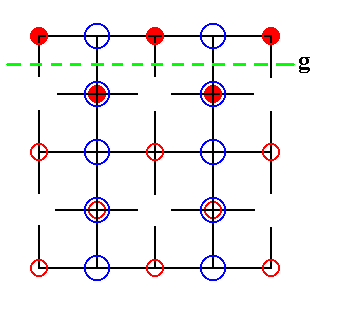Figure 25.   The Sodium ions are still ordered according to glide lines. One such glide line is indicated.

```
```
If we now shift alternative rows of Sodium ions, then we have also eliminated the translations involved in the glide lines between the rows of Sodium ions.
See next Figures.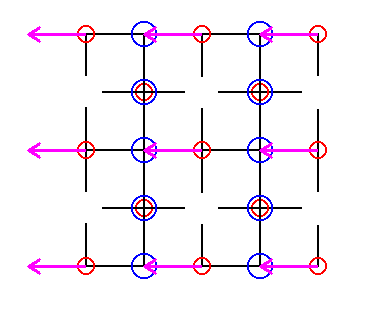Figure 26.   In order to eliminate the translations involved in the glide lines between the rows of Sodium ions, we will shift every second row of Sodium ions as indicated.

```
```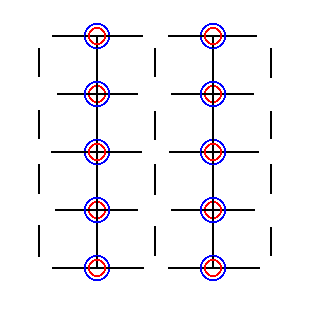Figure 27.   The shifts as specified above finally result in the superposition of the Sodium and the Chlorine ions. Superposition of a Sodium ion and a Chlorine ion means that the centers of both ions coincide. Of course such a superposition (as also the elimination of translations) is just a  c o n c e p t u a l  state of affairs. It is legitimate because we only consider symmetries.

```
```
The empty spaces cannot represent lattice points because they are not equivalent to the other points (which now are furnished with a Na-Cl pair of ions). So the final structure must look like this :Figure 28.   Part of a lattice of which the points are furnished with superimposed ions.

```
```
In the foregoing we have make shifts of alternative rows of ions to the left. But because the structure must be imagined as to be extended indefinitely in exactly the same way (i.e. the structure, as depicted, must be imagined as to be repeated uniformly), we can extend our last obtained result, and get a two-dimensional extended lattice of which all its points are furnished with Sodium-Chlorine pairs of ions -- the members of every such pair are superimposed upon each other. This we've done in the next Figure.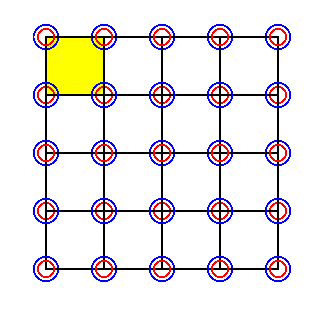Figure 29.   Extension of the pattern in Figure 28. As such it is a 2-D lattice of which the lattice points are occupied with superimposed ions. A unit cell is indicated (yellow). The pattern must be imagined to be extended indefinitly in 2-D space.

```
```
In order to further elucidate the above considerations, we take a somewhat larger section of the NaCl lattice structure and execute both shifts, i.e. the shifts of alternative Cl rows and the shifts of alternative Na rows, at the same time. These shifts eliminate all glide translations.Figure 30.   Elimination of all glide translations by means of shifting the chemical motifs as indicated by the pink and green arrows.

```
```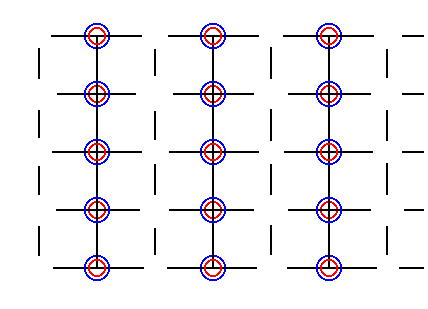Figure 31.   Result of the elimination of all glide translations by means of shifting the chemical motifs as indicated in Figure 30.

```
```
As has been said the open spaces we see in Figure 31, resulting from the shifts, cannot be lattice points, because all lattice points must be equivalent. So the final pattern must look like this (it is of course fully equivalent with the pattern we had already obtained (Figure 28)) :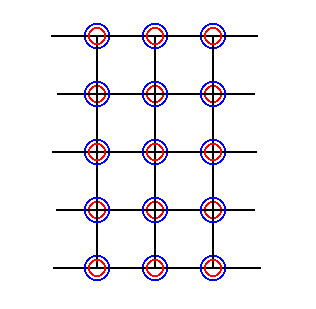Figure 32.   Final pattern as a result of the elimination of all glide translations by means of shifting the chemical motifs as indicated in Figure 30. An extension of this pattern is identical to that of Figure 29.

```
```
If we isolate the unit cell (that was indicated in Figure 29), which means that we eliminate all simple translations, we get the following :Figure 33.   The unit cell of the pattern of Figure 29.
It consists of 4 x 1/4 Cl ion + 4 x 1/4 Na ion, which is equivalent to the superposition of just one Cl ion and one Na ion.

```
```
The symmetry of this 2-dimensional unit cell is 4 m m, which means that it has one 4-fold rotation axis going through its center and perpendicular to the plane of the drawing, further it has two non-diagonal mirror lines and two diagonal mirror lines. It consists of 4 x 1/4 = one Chlorine ion, and 4 x 1/4 = one Sodium ion superimposed upon it. If we do not wish to involve things like "1/4 ion" to figure in our discussion, then we can take the t o t a l   m o t i f , i.e. the complete surroundings of a single lattice point. These surroundings are equivalent to the corresponding unit cell.
See next Figure.Figure 34.   The pattern of Figure 29 with total motifs, and one unit cell indicated.
The unit cell consists of 4 x 1/4 Cl ion + 4 x 1/4 Na ion, which is equivalent to the superposition of just one Chlorine ion and one Sodium ion. It further consists of an amount of  m o t i f  b a c k g r o u n d  having the size of the unit cell.
A total motif ( = complete surroundings of a lattice point) consists of one (whole) Chlorine ion + one (whole) Sodium ion. Further it consists of an amount of motif background having the size of the unit cell.

```
```
In Figure 34 we see the complete equivalence of total motif and unit cell. Also their symmetry is identical :Figure 35.   The total motif and unit cell of the pattern of Figure 34. Both possess a symmetry of 4mm.

```
```
If we don't want the  s h a p e  of the  m o t i f  b a c k g r o u n d  ( = the complete surroundings of a lattice point) to play such a decisive role in determining the symmetry, and if we also don't want to involve  p a r t s  of ions, we can choose a larger total motif and a corresponding larger unit cell. The next Figure shows such a larger unit cell choice, but we still end up with  p a r t s  of ions :Figure 36.   Choice of a larger total motif (light blue) and (correspondingly larger) unit cell (yellow) of the pattern of Figure 34. Now the dark colored points are the lattice points (nodes) of a square net (and this is a net without centerings). The unit cell is indicated in yellow. Below it a same unit cell is outlined, showing the fact that now the unit cell consists, as in the former case, of four motifs, but whereas in the former case each motif consisted of 1/4 Na + 1/4 Cl ions, in the present case each motif consists of 4 x 1/4 Na + 4 x 1/4 Cl ions (Alternatively, we can stick to the original lattice, but then the unit cell is just a multiplication of a smaller unit cell -- the original unit cell -- and is not a genuine unit cell, i.e. not the smallest possible repeat unit having the shape of a square).

```
```
The new net (square lattice), established in Figure 36, is emphasized (bold black lines) in the next Figure.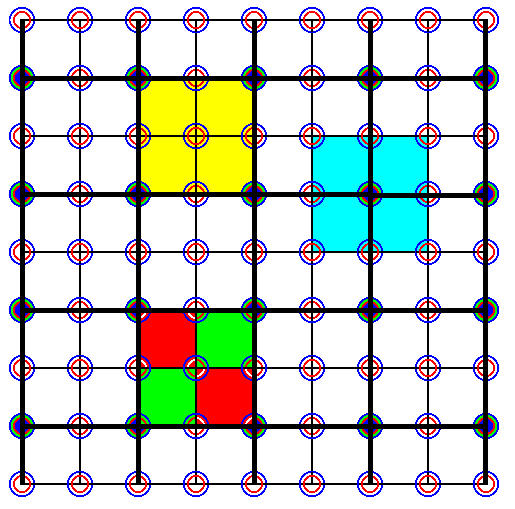Figure 36a.   The same as Figure 36, but now the new net (square lattice) -- as a result of the choice of a larger unit cell -- emphasized by thick black lines.

```
```
In the next Figure the lines of the old net are erased. The new net is now clearly indicated.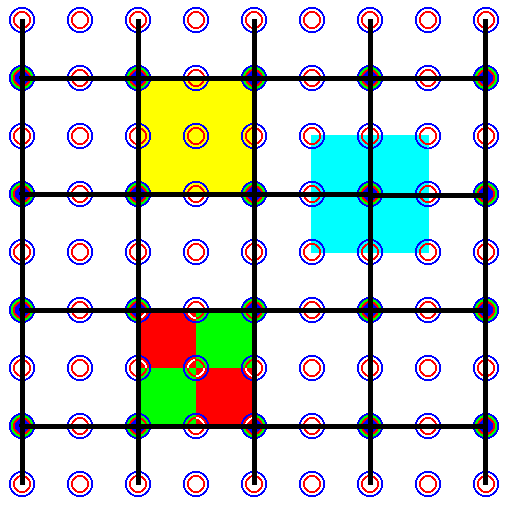Figure 36b.   The same as Figure 36a, but now with the lines of the old net erased.

```
```
Although the symmetry of the content of the unit cell (i.e. the symmetry not only of the unit cell as empty building block, but as furnished with motifs) and the symmetry of the total motif is now not determined solely by the shape of the motif background anymore, but by the distribution of Na--Cl ion pairs, the above unit cell choice, and also the corresponding total motif, still contains  p a r t s  of ions.
The next Figure shows how to obtain a unit cell, and a total motif, such that they contain only  w h o l e  ions. Moreover, in contradistinction to what we had found in Figure 35, the unit cell, and also the total motif, consists of more than one superimposed ion pair, guaranteeing that the symmetry of the content of the unit cell, and also the symmetry of the total motif, is not solely determined by the shape of the motif background. Now the positioning of the four Na--Cl ion pairs determines a definite pattern and thus a definite symmetry (While one such pair, as it is in itself, must be considered as (a) spherical (object), and thus possessing maximal symmetry, implying that it does not limit, i.e. not (further) determine the symmetry of the content of the unit cell or the symmetry of the total motif).Figure 37.   This choice of a larger total motif and (correspondingly larger) unit cell of the pattern of Figure 34 yields a unit cell and a total motif containing only  w h o l e  i o n s.  The intersections of the green lines were the original lattice points. Now the intersections of the black lines are considered as lattice points instead of the original ones. A possible unit cell is indicated in yellow. Also a total motif is indicated (light blue) in relation to the corresponding unit cell (yellow). Such a unit cell (and total motif) is, however, not the smallest one possible (while having the shape of a square). It can nevertheless be chosen as a unit cell, if things are demanding such a larger unit cell, as is indeed the case. We can, however, adapt the lattice, i.e. change our assessment of lattice points, in such a way that our unit cell becomes a genuine unit cell with exactly the same content. The corresponding adaptation of the present Figure will then look like the image of the next Figure.

```
```Figure 37a.   Establishment of a new lattice accommodating the unit cell as indicated in Figure 37. The unit cell is indicated in yellow. A total motif (light blue) is indicated in relation to the corresponding unit cell. The intersections of the black lines are the lattice points (nodes). A unit cell consists of four pairs of superimposed Na--Cl ions ( + motif background). A total motif also contains four pairs of superimposed Na--Cl ions + motif background. The indicated total motif (light blue) is associated with the upper right lattice point of the corresponding unit cell ( both unit cells, depicted in the present Figure (yellow), are of course totally equivalent ).

```
```
The next Figure isolates the unit cell (or total motif for that matter) found in Figure 37 and 37a. This isolation is equivalent to the elimination of all the  s i m p l e  translations (responsible for the repetition of the unit cell). As such this unit cell is the sought for Complex Motif, but still of a two-dimensional version of NaCl, i.e. of one single layer (slice, sheet) of the true NaCl structure.Figure 38.   The Complex Motif of the two-dimensional version of NaCl. It consists of four NaCl units. Its symmetry is that of a Square, 4mm. (In 3-D it corresponds to a Cube).

```
```
Going three-dimensional
In all the foregoing we have considered  o n e  s i n g l e  l a y e r  of the NaCl structure.
When we do the same with all the other parallel layers, we end up with a stack of layers (and this whole is now three-dimensional), layers, that are identical to each other. They consist of equally spaced Na-Cl pairs. Within each layer these pairs are separated by a distance which is half the simple translation involved in the repetition of the NaCl cubic unit cell (as depicted in Figure 15a). Because this distance is the smallest distance at all involved in our discussions, the layers must be precisely aligned to each other, meaning that the motifs of the upper layer lie exactly above those of the layer directly beneath, while the motifs of the latter in turn lie exactly above those of the layer directly beneath it, etc. So by repeating the above actions (shifts, etc.) in all the successive (say, horizontal) layers of the NaCl structure, parallel to the initial one, we will obtain the situation for three dimensions, and in this way we will end up with a unit cell that has the full symmetry of a  c u b e. See next Figure.Figure 38a.   Four images of the Complex Motif of a crystal of (three-dimensional) Sodium Chloride (NaCl). It is also a unit cell, derived from the 2-D unit cell of Figure 38. It consists of  e i g h t  NaCl units + motif background. Its symmetry is that of a Cube (regular hexahedron), 4/m 3* 2/m.
Upper left image :   The eight NaCl units as seen from above or below.
Upper right image :   The same eight NaCl units as seen from front or rear.
Lower left image :   The same eight NaCl units as seen from left or from right.
Lower right image :   The same eight NaCl units as seen from front, from right and from above.

```
```
The arrangement of the NaCl units within the (cubic) unit cell is itself also an arrangement according to the geometry of a  c u b e  (which can be inscribed in the unit cell), each corner of which is occupied by one Chlorine ion superimposed upon one Sodium ion. Because the very  a r r a n g e m e n t  (pattern) of the NaCl units (within the newly established unit cell) IS our sought for (3-D) Complex Motif, we can concentrate on it (and forget about any unit cell). The symmetry of this arrangement is 4/m 3* 2/m, and thus we have ended up with a Complex Motif -- that resulted after the elimination of the translations involved in the glide planes, and finally, of all simple translations -- which has the same symmetry as the unit cell we started with in the first place :   the unit cell depicted in Figure 15a. So indeed the elimination of the translations involved in the glide planes of the NaCl structure does not increase the symmetry any further. This was to be expected because the 4/m 3* 2/m symmetry is not only the highest possible symmetry of the Isometric Crystal System (in which NaCl crystallizes), but also the highest symmetry possible in crystals at all.

The next Figure depicts this Complex Motif as a pattern of eight NaCl units. The cube indicates the arrangement of those units, each of which is a Na--Cl pair (The members of such a pair must be imagined as to be superimposed upon each other, in such a way that their centers coincide). The horizontal and vertical distances that separate these motif units are half the distance that originally separated the Chlorine ions from each other or, equivalently, that separated the Sodium ions from each other (This distance is the translation distance involved -- as simple translation -- in the repetition of the original unit cell, and that means the unit cell as depicted in Figure 15a (or 15 for that matter)). Each NaCl unit must be imagined as a sphere (in fact as two concentric spheres).Figure 39.   The Complex Motif of (three-dimensional) NaCl. It consists of eight NaCl units. Such a unit is -- in the present case -- ( as is the whole Complex Motif ), just a  c o n c e p t u a l  e n t i t y,  it consists of an Na ' ion ' on which is superimposed a Cl ' ion ' in such a way that their centra coincide. The cube + other auxiliary lines only serve to indicate the three-dimensional arrangement of the eight NaCl units, together making up the Complex Motif.

```
```
Because this Complex Motif consists of  e i g h t  equal motif units, each at a same distance from a center, we can assess the geometry of the pattern of motif units, representing the Complex Motif, as a pattern possessing  e i g h t  a n t i m e r s.  These antimers are congruent and are regularly positioned around the center of the whole pattern. This means that the promorph or stereometric basic form must belong to the regular endospheric polyhedrons or Polyaxonia rhythmica. In that promorphological category we have to do with regular (endospherical) bodies bounded by faces. Each of these faces can be seen as the base of a pyramid. These pyramids come together with their tips in a central point -- the center of the polyhedron -- and represent antimers. Such an antimer itself represents a body part (where "body" now stands for that  particular r e a l  body or pattern of which we assess its promorph) that is regularly, but non-periodically, repeated around a point, an axis or a plane. In our case -- the Complex Motif (considered as a real pattern) of single non-twinned crystals of Sodium Chloride (NaCl) -- we have to do with eight congruent antimers positioned around a  p o i n t  which is the center of that Complex Motif. So the regular polyhedron representing this situation must be the  R e g u l a r  O c t a h e d r o n,  which has eight congruent (triangular) faces. Each of these faces is the base of a regular pyramid pointing inwards. There are eight such pyramids coming together with their tips in the center of the octahedron. They represent the eight antimers (themselves represented by the eight Na--Cl pairs). So the promorph of any single non-twinned NaCl crystal must belong to the Octaedra regularia (Polyaxonia).
The motif units of our Complex Motif, as depicted in Figure 39, are arranged according to the vertices of a cube. The next Figure illustrates the close kinship between a cube (hexahedron) and a regular octahedron.Figure 40.   A regular octahedron can be inscribed in a cube. The Figure shows how the eight motif units, as situated at the corners of a cube as in Figure 39, can be associated with the eight faces of the octahedron, or better, with the eight pyramids (that have those faces as their base) that constitute the octahedron.

```
```
Because the symmetry of the original cubic unit cell, as depicted in Figure 15a, was not increased by the eliminations of the glide translations, we could also take this original cubic unit cell as representing the Complex Motif. However, in doing so a disadvantage is involved which consists in the fact that we then have to do with  p a r t s  of ions, which is theoretically legitimate, but not very intuitive and so not very satisfactory. So our long derivation of the Complex Motif by eliminating the glide translations was not superfluous after all. See next Figures.Figure 41.   The repetition of the original unit cell (Figure 15a), demonstrated in one sheet of the NaCl structure. We can see that the unit cell is exactly repeated across the structure. However, in addition to one whole ion, each unit cell sheet also contains  p a r t s  of ions.

```
```Figure 42.   If we try to select a unit cell (also containing more than one Na and more than one Cl), such that no  p a r t s  of ions are involved, we see that it does not repeat exactly. So it is not a unit cell, nor a total motif, and consequently we cannot interpret it as a complex motif.

```
```Figure 43.   If we select a total motif corresponding to the unit cell of Figure 41 ( or, equivalently, if we consider it as a unit cell ), we see that it contains to be sure only whole ions, and it further repeats exactly, but its symmetry content is not representing all symmetry content which a NaCl crystal has in store. So also this cell is not suited to represent the Complex Motif ( The latter must show all point symmetry that the crystal has in store ).

```
```Figure 44.   If we select a larger unit cell than the one in Figure 42, we still do not get what we want :   The cell is indeed exactly repeated across the structure, but it does not show all the point symmetry that a NaCl crystal has in store.

```
```
So if we reject  p a r t s  of ions to play a role in the constitution of the Complex Motif, and if we further demand that the symmetry is determined by a  p a t t e r n  of ions (and not solely by the shape of the motif background), and, finally, if we demand that the Complex Motif must possess  a l l  t h e  p o i n t  s y m m e t r y  that the NaCl crystal has in store (implying that of each ion species at least six individuals (in octahedral coordination) must take part in the constitution of the Complex Motif -- in fact eight were needed), then we are left with acknowledging the above derived Complex Motif (Figures 38a and 39) as the only one possible.
Of course the  s y m m e t r y  of the Complex Motif was already given by the original unit cell (Figure 15a). To reveal the Complex Motif itself was necessary in order to decide whether the promorph of an NaCl crystal belongs to the Hexaedra regularia or to the Octaedra regularia, which both involve the same symmetry, but differ in their number of antimers.

This concludes our exposition of the determination of the Complex Motif of single non-twinned crystals of Sodium Chloride.

In the next document we will do the same with some other crystalline substances.

```
```
To continue click HERE to proceed further with the theoretical preparation to the promorphology of 3-D crystals (third part).
```

```
```
```
e-mail :back to The Thermodynamics of Crystals

back to Tectology

back to Introduction to Promorphology

back to Anaxonia, Homaxonia, Polyaxonia

back to Protaxonia : Monaxonia

back to Stauraxonia homopola

back to Stauraxonia heteropola

back to Homostaura anisopola, Heterostaura

back to Autopola oxystaura and orthostaura

back to Allopola (introduction)

back to Allopola amphipleura and zygopleura

back to Spiraxonia

back to Spiraxonia allogonia

back to Spiraxonia isogonia

back to the Basic Forms of Cells I

back to the Basic Forms of Cells II

back to the Basic Forms of Organs

back to the Basic Forms of Antimers

back to the Basic Forms of Metamers

back to the Basic Forms of Persons

back to the Basic Forms of Colonies

back to the first part of the Preparation to the Promorphology of Crystals

back to the second part of the Preparation to the Promorphology of Crystals

back to the third part of the Preparation to the Promorphology of Crystals

back to the fourth part of the Preparation to the Promorphology of Crystals

back to the fifth part of the Preparation to the Promorphology of Crystals

back to the sixth part of the Preparation to the Promorphology of Crystals

back to the seventh part of the Preparation to the Promorphology of Crystals

back to the eighth part of the Preparation to the Promorphology of Crystals

back to the ninth part of the Preparation to the Promorphology of Crystals

back to the tenth part of the Preparation to the Promorphology of Crystals

back to the eleventh part of the Preparation to the Promorphology of Crystals

back to the twelfth part of the Preparation to the Promorphology of Crystals

back to the thirteenth part of the Preparation to the Promorphology of Crystals

back to the fourteenth part of the Preparation to the Promorphology of Crystals

back to the fifteenth part of the Preparation to the Promorphology of Crystals

back to the sixteenth part of the Preparation to the Promorphology of Crystals

back to the seveneenth part of the Preparation to the Promorphology of Crystals

back to the first part of the Preparation to the Promorphology of 3-D Crystals

****************